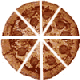# Circumference and Area of Circles

Circumference and area of circles is presented in this unit. Students will also learn about the parts of a circle. Try our sample lessons below, or browse other units of instruction.Circumference and Area Description Geometry and the Circle To define and identify points, line segments, planes, diameter, radius and chord. Circumference of Circles To define circle, Pi and circumference. To understand that Pi is a constant for any circle. To find circumference of a circle using the proper formula. To relate circumference to real-life objects. Area of Circles To find the area of a circle using the proper formula. To find the radius or diameter given the area. To relate area to real-life objects. Practice Exercises To complete 10 additional exercises as practice. To assess students' understanding of all circumference and area concepts learned. Challenge Exercises To solve 10 additional problems that challenge students' understanding of circumference and area. Word problems connect math concepts to the real world. To hone students' problem-solving skills. Solutions To review complete solutions to all exercises in this unit. Includes the problem, step-by-step solutions, final answer and units for each exercise. Learning Objectives Learning Topics: Geometry of a Circle, line segment, chord, plane, radius, diameter, pi, Circumference of Circles, and Area of Circles.